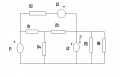# Need help with finding one current in circuit with a lot of elements with unknown values.

#### cdummie

Joined Feb 6, 2015
124
In the circuit shown in the picture, current I is known and it's I=-80mA if E1=10V, if E1=38V current I is I=40mA. Find the I if E1=17V. Known values are: E2=6V, R1=300Ω and R5=100Ω.This is what i have:

I tried using linearity theorem (i believe that's the correct name of the theorem):

I=E1*a+b

-80=10a+b first case
40=38a+b second case

from this equations i can find a and b

80=-10a-b
40=38a+b

120=28a a=4.29

now into first equation

-80=42.9+b b=-122.9

now i have a and b for the third case:

I=E1*a+b

I=17*4.29-122.9

I=-49.97mA

Is this correct, and is this good approach?

#### WBahn

Joined Mar 31, 2012
26,398
Look good, except you need to learn to use units properly.

As a check, you are going 7V of the 28V it takes to get from 10V to 38V. So that's 1/4 of the way. Hence the current should change by 1/4 of the way from -80mA to 40mA. Does it?

#### cdummie

Joined Feb 6, 2015
124
Look good, except you need to learn to use units properly.

As a check, you are going 7V of the 28V it takes to get from 10V to 38V. So that's 1/4 of the way. Hence the current should change by 1/4 of the way from -80mA to 40mA. Does it?

Well since 1/4 of difference between I in first case and I in second case is 120mA/40 then it should increase by 30mA and it should be -50mA, since i got 49.97mA it is close enough, thank you for showing me this, it's a good way to check if made a mistake while solving equations.#### WBahn

Joined Mar 31, 2012
26,398
Yep.

You want to get in the religious habit of always, always, ALWAYS asking:
1) Do the units work out (which is only possible if you properly track them)?
2) Does the answer make sense?
3) Does the answer check out (i.e., is it really a solution to the problem)?

Most mistakes you make will result in at least one of these failing. That means you can catch mistakes before you submit them. In school that will significantly boost your grades while in the real world it will significantly boost your employability while significantly decreasing the likelihood that an uncaught mistake will kill someone someday.

•Saviour Muscat and cdummie